# Prospect and Competence of Quantitative Methods via Real-time PCR in a Comparative Manner: An Experimental Review of Current Methods

Hossein Mahboudi1, Negin Mohammadizadeh Heidari2, Zahra Irani Rashidabadi2, Ali Houshmand Anbarestani3, Soroush Karimi4, Kaveh Darabi Darestani5, *
1 Department of medical biotechnology, School of Advanced Technologies in Medicine, Shahid Beheshti University of Medical Sciences, Tehran, Iran
2 Department of Agronomy and Plant breeding, Agricultural Faculty, Zanjan University, Zanjan, Iran
3 Department of Genetics, Faculty of Biological Sciences, Tarbiat Modares University, Tehran, Iran
4 Nano Drug Delivery Research Center, Kermanshah University of Medical Sciences, Kermanshah, Iran
5 Biology Department, School of advanced sciences regenerative medicine, Tehran Medical Branch Islamic Azad University, Tehran, Iran

open-access license: This is an open access article distributed under the terms of the Creative Commons Attribution 4.0 International Public License (CC-BY 4.0), a copy of which is available at: (https://creativecommons.org/licenses/by/4.0/legalcode). This license permits unrestricted use, distribution, and reproduction in any medium, provided the original author and source are credited.

* Address correspondence to this author at the Biology Department, School of advanced sciences regenerative medicine, Tehran Medical Branch Islamic Azad University, Kaveh Darabi Darestani, Tehran, Iran; Tel: +989191858816; E-mail: kavehdarabi@ut.ac.ir

## Abstract

### Background:

There are numerous approaches dealing with relative and absolute quantitation. The methods differ in their efficiency assumption and applicability.

### Objective:

Current methodologies and rations used in qPCR quantification were compared in an experimental study of transgenic copy number determination of a monoclonal antibody Daclizumab.

### Methods:

With an inter and intra-methodical view, variations in relative and absolute quantification strategies were discretely extracted and compared to one another.

### Results:

In relative quantification, six methods were studied and the ratios were computed relative to Glucagon as internal control. For Absolute quantification, the calculations were based on standard curve. Relative quantification considers the relative changes in expression levels while Absolute quantification relates the PCR signal to input copy number with a calibration curve.

### Conclusion:

The observed unevenness of the ratios in Relative approach pointed mainly to the efficiency changes and its calculation formula. Whereas results in Absolute approach strategies showed homogeneity which indicates the consistency of the calculation method.

Keywords: Absolute, Relative, Copy number, qPCR, Quantification, Monoclonal, Daclizumab.

## 1. INTRODUCTION

One of the demanding methods to quantify gene expression is Real-time Polymerase Chain Reaction [1-5]. The technique quantifies the target genomic region and at the same time holds the detection potential.

Despite the applicability of old quantitation methods (i.e.Southern blotting) to measure transgene copies, the shortages appear while dealing with a large number of samples  along with other obstacles like being time-consuming, involving substantial amounts of DNA from samples and using harmful radioisotopes in some instances . Profound, and operational quantitative methods based on the PCR technique, including Competitive PCR  and real-time PCR methods were improved to solve these difficulties [6, 7, 9].

The logic behind qPCR is detecting the amount of the amplified genomic product in real time by means of various chemicals (e.g.specific DNA binding dyes, hydrolysis probe, molecular beacon etc.), which leads to creation of a florescent sigmoid curve . There are main steps in determining copy number via qPCR, including: choosing the appropriate genomic region, choosing specific primers, practical validation of the primers, making accurate serial dilution of genomic DNA, and finally selecting an appropriate detecting chemicals . Considering all the prosperous applications of qPCR for gene copy number calculations, there are some aspects of the method, which raise concern regarding its accuracy . Depending on the purpose of the study, two different methods, Absolute and Relative quantification, for evaluating data from quantitative PCR can be used. By linking the PCR signal to a standard curve, Absolute quantification expresses the copy number of the transcript of target or control gene (i.e.defining expression stages in absolute numbers of copies). Relative quantification designates the variation in expression of the target gene relative to some reference group [10, 13-16]. It means showing fold changes in expression between two samples.

The aim of the present study was to determine the genetic stability of recombinant monoclonal antibody Daclizumab in the host CHO cell line at DNA level that includes copy number determination of the Daclizumab gene by Real-time PCR technique via various relative and absolute quantification approaches.

## 2. MATERIALS AND METHODS

### 2.1. Genomic DNA Isolation

Genomic DNA of the recombinant Daclizumab producing CHO Cell pellets was extracted using genomic DNA extraction kit (GeneJET Genomic DNA purification kit, Thermo scientific, Massachusetts, United States) according to the manual. For testing the quality of the extracted DNA as well as the plasmids of Daclizumab and Glucagon, 5 µl of each sample was run on a 1% agarose gel with a 1kb ladder. The total amount of extracted DNA was measured by spectrophotometer at wavelength of 260 nm.

### 2.2. Cloning

Cloning kit containing pTG19-T PCR cloning vector and T4 DNA ligase, was purchased from Vivantis (Malaysia). DH5-α E. coli strain was used as a bacterial strain for cloning. Partial Daclizumab and Glucagon gene sequences were cloned into separate pTG19-T vectors according to manufacturer manual.

Plasmid copy number and stock solution preparation

Copy number of each plasmid (per microliter) was calculated based on equation 1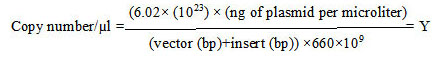(Equation 1)

Stock preparation of each plasmid (109 copy/µl) is obtained based on Y from the aforementioned formula and Equation 2.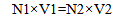(Equation 2)

109 (copy/µl) × 100 µl = Y (copy/µl) × X (µl)

Equation 2 indicates that for preparation of 100 µl of 109 copy/µl stock plasmid, X microliter of the stock is reached to a volume of 100.

### 2.3. Standard Solutions Preparation

A series of standard solutions containing 107, 106, 105,104,103, and 102 plasmid copies/µl were prepared by making a serial dilution of the concentrated target or reference gene stock solutions (109 copy/µl), respectively.

### 2.4. Quantitative Real-Time PCR

Glucagon was used as the internal control gene [18, 19]. The two amplification primers were selected in appropriate regions of Daclizumab, and Glucagon genes. The primer sets were designed so that the length of amplicons would be the same Table (1).

Table 1. List of primers and their specifications used for qPCR analysis.
Gene Primer Forward 5´-3´ Primer Reverse 5´-3´
Glucagon GTGAGCAGGGTATCTGTGGTTTGG TACAGCACCTTCTGAGAGCATCCA
Daclizumab TACTAGCTACAGGATGCACTGGGTAAG GACTGCGGTGTCCTCAGATCTCAG

Maxima SYBR Green qPCR master mix was purchased from Thermo Scientific. For the reactions in each tube, 50 ng of the extracted genomic DNA (or nuclease-free water for testing no template control or NTC) in triplicate and 1 µl of the prepared standard solutions were used Table (2A & B) as template for Real-time PCR.

Table 2. Reagents and solutions for the qPCR reaction in study sample (A) and standard (B).
A
>Master Mix (2X) >7.5 µl
Template 50 ng
Primer Mix (10x) 1.5 µl
Nuclease Free Water up to 15 µl
Total Volume 15 µl
B
Master Mix (2X) 7.5 µl
Standard Dilution 1 µl
Primer Mix (10x) 1.5 µl
Nuclease Free Water 5 µl
Total Volume 15 µl

Real-time PCR was performed on the Corbett Rotor-Gene 6000 (Rotor-Gene 6000 software version 1.7.87). Run was performed as a 2-step protocol with an initial hold of 95 ºC for 10 min, followed by 50 cycles of a denaturation step at 95 ºC for 30s, annealing at 58 ºC for 20s and an extension at 72 ºC for 20s. Raw data were processed by the Analysis-Only Rotor-gene Q series software (Corbett) using the settings baseline subtraction and linear regression, to obtain the Ct (Cycle Quantification)  values.

### 2.5. Quantification Methods: Relative and Absolute Strategies

Copy number of the Daclizumab gene was calculated via the obtained Ct values for Daclizumab and Glucagon genes from genomic DNA sample based on the slopes and intercepts of the constructed standard curves. For a comprehensive comparison amongst formula and rations used in Relative and Absolute quantification strategies, some relevant mathematical methodologies were employed. For relative quantification, either 100% efficiency for all runs or the actual calculation was considered.

### 2.6. Relative Methods

After measurement of Ct values, various methods can be used to conclude the expression level of the target gene in the test sample relative to the calibrator sample.

### 2.7. The 2-ΔΔCt (Livak) Method

This method, which is extensively used and easily performed, considers the probability that both target and reference genes are amplified with similar efficiencies near 100%. Prior using the Livak method (Equation 3), it is crucial to validate these assumptions by determining the amplification efficiencies of target and the reference genes .

ΔCt(test) = Ct (target, test) - Ct (ref, test)

ΔCt(control) = Ct (target, control) - Ct (ref, control)

ΔΔCt= ΔCt(test)- ΔCt(control)(Equation 3)

One sample efficiency corrected calculation models

One procedure of calculation of the relative quantification ratio is the efficiency corrected calculation model, based on one sample (Equations 4–5)  LightCycler Relative Quantification Software, 2001).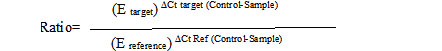(Equation 4)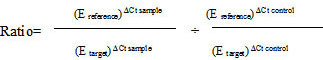(Equation 5)

### 2.8. The ΔCt Method Using a Reference Gene

The ΔCt method using a reference gene is a variant of the Livak method that is easier to execute and gives basically the identical results.

Ratio (reference/target) = 2Ct(ref) - Ct(target)

The mathematical assumptions for this approach are identical to those for the Livak method.

LinRegPCR

A different way to analyze qPCR efficiencies is based on mathematical operations that calculate the efficiency for each single fluorescence curve. To do this, an altered formula derived from the equations in  was used (Equation 6).

Relative transgene copies= 2-ΔCt

Ratio= 2-ΔΔCq

E= 10 (-1/slope)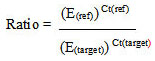(Equation 6)

### 2.9. Calculation of Number of Transgene Copies

By placing the mean Ct value (after ruling out the out-of-range Ct values) and the numbers deduced from the standard curves in the formula, the copy number of Daclizumab gene was calculated according to the following formula (Equation 7):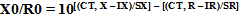(Equation 7)

In the aforementioned formula IX and IR stand as intercepts of the relative standard curves of target and reference genes, separately, SX and SR remain as the slopes of the standard curves of target and reference genes, correspondingly, and CT, X and CT, R are the distinguished threshold cycles of the amplification of the target and reference genes to an established sample. If the copy number of the reference gene (R0) is well confirmed, the copy number of the target gene (X0) can easily be deduced from the IX, IR, SX, SR, CT, X, and CT, R in the tested sample .

### 2.10. Absolute Quantification

The Ct values assumed by the following equation were employed to calculate the logarithm of the recombinant gene copy numbers from:

Ct= slope×log (GCN)+I

where I in the formula above acts as the intercept of standard curve . Another way to obtain the absolute copy number is referring to calculated values by the Real-time PCR device. However, the following conditions must be satisfied prior any judgement regarding the obtained values.

The average value of the slope of the standard curves should be in the range of - 3.1 ≤ slope ≤ - 3.6, corresponding to amplification efficiencies of 110% to 90%. The values of R2 in the standard curves have to be ≥0.98. The limit of quantification (LOQ) should be ≤ the lowest amount or concentration included in the dynamic range (i.e.0.09% or 50 copies). For combined modules the limit of detection (LOD) should be < 0.045% with a level of confidence of 95%, ensuring ≤ 5% false negative results.

A sample was considered negative when no Ct value was determined and no amplification curve was observed.

A sample was considered positive when Ct value was less than 45 and amplification curve was observed.

For test items, if any inconsistent result was achieved the test was repeated.

## 3. RESULT

Relative Quantifications

The results are presented as mean values from three biological samples analyzed in triplicates. For NTC, no Ct values was recorded in Real-time PCR output. The obtained mean Glucagon and Daclizumab Ct values (in triplicate) were 23.34±0.11 and 18.20±0.14 respectively.

### 3.1. Calculation of Amplification Efficiency

A five step 1:10 dilution series was organized and each dilution step was measured in triplicate Table (3).

Table 3. Daclizumab and Glucagon amplification efficiency (E) calculation using means (n = 5) of calibration curves generated via dilution series of plasmid of each gene.
- Daclizumab Glucagon
Dilution Ct %Var Ct %Var
107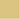13.97 0.43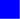15.56 0.61
106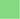17.32 0.66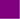18.93 0.75
105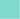21.33 0.73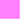22.33 0.93
104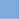24.61 0.34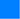26.08 0.82
103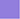27.20 0.56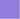29.12 0.57
- Slope E I Slope E I
- -3.374 1.976 37.759 -3.425 1.958 39.533

Relative fluorescence curves of Daclizumab and Glucagon plasmids plotted against the cycle plus the relevant standard curve which was used for calculations of efficiency, slope and intercept were prepared Fig. (1). To check the fidelity of the amplified samples and consequent curves, melt A. green analysis was generated for the target and reference gene Fig. (2).

### 3.2. Relative Transgene Copy Numbers

The data set applied to calculate the relative gene copy numbers is presented in Table (4). The transgene copies were relative to Glucagon using the analyzed Ct values, slope, intercept and the qPCR efficiencies.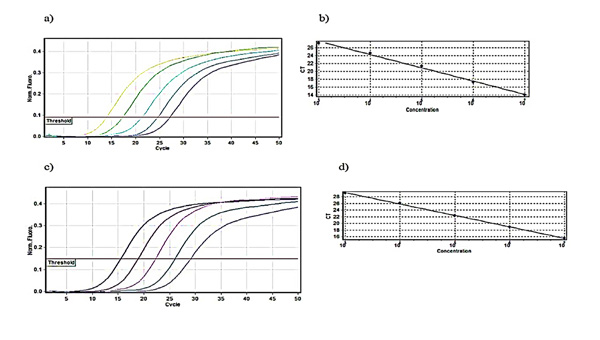Fig. (1). Relative fluorescence curves plotted against the qPCR cycles (A & C) plus standard curve showing Ct plotted against concentration (B & D) are shown for Daclizumab and Glucagon plasmids serial dilutions as standard respectively. Colors corresponds to those in Table 3.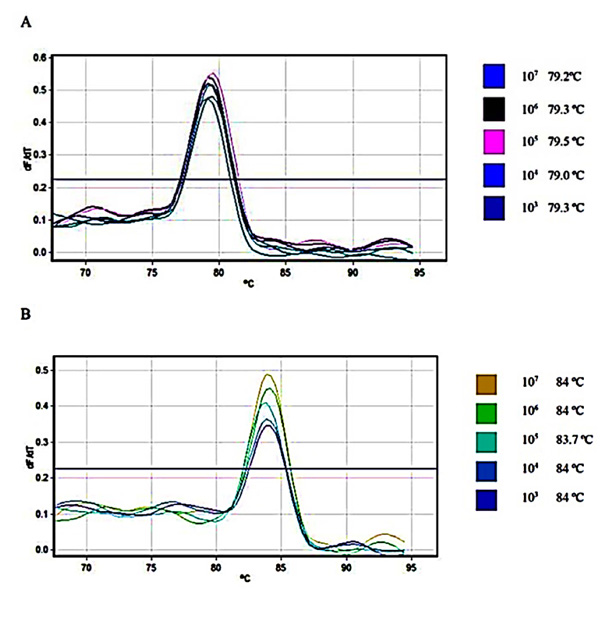Fig. (2). Melt data for Melt A. Green analysis showing peaks for each standard dilution series in Glucagon (A) and Daclizumab (B).

Table 4. Calculation of gene copies relative to Glucagon via different relative methods.
Relative Quantitation Method 2-ΔΔCt (Livak) One Sample Efficiency Corrected Formula 1 One Sample Efficiency Corrected Formula 2 The ΔCt Method LinRegPCR Number of Transgene Copies 
Daclizumab-Glucagon
Efficiency 2-2 1.976-1.958 1.976-1.958 2-2 1.882-1.864 NO
RGCN±Var 17.63±0.15 16.68±0.13 16.48±0.11 35.26±0.12 30.72±0.11 11.74 ±0.18
GCN: Relative Gene Copy Number Var: Variance NO: Not operated in the formula

The results acquired from the diverse calculation methods expressed that the ΔCt method for efficiency correction resulted in the highest relative transgene numbers followed by LinRegPCR, 2-ΔΔCt, one sample efficiency corrected equation 4, one sample efficiency corrected equation 5 and Number of transgene copies .

### 3.3. Absolute Quantification

The absolute gene copy number of both target and reference gene was compared (in duplicate) with the calculated results obtained from Real-time PCR software Table (5).

Table 5. The obtained absolute quantification results via standard curve for Glucagon and Daclizumab genes by means of formula and qPCR device output.
Gene Ct Formula (Edros et al., 2013) Device Calculation (Copies/reaction)
Glucagon 1 24.82 19952 19671
Glucagon 2 24.72 21379 21131
Daclizumab 1 19.27 301995 19671
Daclizumab 2 19.40 275422 276114

## 4. DISCUSSION

Nucleic acid quantification is an applicable issue for the description of mammalian recombinant cell lines and also for the recording of producer clones . Quantitative real-time RT-PCR is broadly and progressively used in different kinds of gene quantification strategies, due to its wide range of dynamic quantification, satisfactory reproducibility and great thoroughness. Whereas, qPCR has an incredible potential for analytical applications, an ample understanding of its underlying principles is essential. Real-time PCR data are calculated relatively and absolutely.

In the present study, different approaches on the relative quantification of target gene transcripts in comparison to an internal control gene transcript plus two sources of absolute quantification were investigated and compared. The mathematical models for data analysis were presented to calculate the relative expression ratio on the basis of the PCR efficiency and crossing point deviation of the investigated transcripts. Different methods of relative and absolute quantification used in the present study showed variations in the calculated transgene copy number.

### 4.1. Relative Quantification

Relative quantification is based on the assessment between the expression of a target gene versus a reference gene . The method is conducted via the expression levels of a target gene versus a housekeeping gene (reference or control gene) and in theory is suitable for most objectives to inspect physiological variations in gene expression levels. The units employed for expression of relative quantities are unrelated, and the relative quantities can be associated with multiple real-time qPCR experiments. In Real-time PCR analysis, the first crucial step is to acquire the endogenous DNA sequence with established low copy numbers per genome as the internal control. A single-copy endogenous gene per genome is the ultimate choice for this estimation to ensure sensitivity of detection . In our experimental design, Glucagon was chosen as the single-copy or internal control gene. The gene has been shown to be single copy in many studies [e.g 10, 18, 20, 26] especially in mammalian cells. Since the Daclizumab as a monoclonal antibody is produced in recombinant Chinese Hamster Ovary (CHO) cell line, Glucagon could be a great choice as the single copy gene. Regarding the other approach, Absolute quantification employs an internal or external calibration curve to develop the input template copy number . In qPCR analyses, reference genes or internal controls are employed as controls to standardize the data by revisions for differences in quantities of cDNA used as a template. A flawless reference gene is hence one that does not display changes in expression between samples from different experimental conditions or time points. Several genes, such as GADPH, ACTB, or 16S rRNA, are repeatedly applied as reference genes. However, a number of studies have revealed that the expression of these genes may vary substantially between tissues or between treatments, and this inconsistency may make them unsuitable for use as reference genes . So, Reference genes must be cautiously designated based on experimental data. A good reference gene is characterized with an M value below 0.5 in uniform sample sets, and below 1 in heterogeneous sample sets .

To evaluate qPCR quantification methods, six different relative quantification approaches were employed. There are some reasons for efficiency variations apart from calculation assumptions including temperature differences (in plate based machines), the presence of PCR inhibitors and inaccurate pipetting causing less or more volumes of master mix in reactions.

To overcome the problem of varying amount of input template of the target gene, normalizing to a reference gene is a solution . The 2-ΔΔCt method may be employed to compute relative changes in gene expression . For the validation of the ΔΔCt, the amplification efficiencies of the target and reference must be almost identical. A promising aspect of the 2-ΔΔCt method is that it utilizes data as part of the real-time PCR experiment to accomplish this normalization task . The dependency on an incorrect assumption of 100% PCR efficiency plus neglecting background florescence which cause results deviation, are main disadvantages of 2-ΔΔCt method . To solve the issues, individual efficiency corrected calculation is introduced .

The ΔCt method utilizes the difference between target Ct and reference values for each sample. In spite of the easiness of the method, it is certainly normalized expression. The expression level of the reference gene is taken into account. The main difference in the results is that the expression value of the calibrator sample is not 1.0. If the resulting expression values gained in this method are divided by the expression value of a chosen calibrator, the results of this calculation are precisely the same as those obtained with the Livak method. As we did the operation in the present study for the result of the ΔCt method (35.26±0.12), after dividing by the calibrator expression value, a similar result like in 2-ΔΔCt method was gained.

In one sample efficiency corrected model, the expression of target-gene is normalized by one reference gene (REF) expression, which is actually derived from classical and frequently described reference genes [29, 31]. The fundamental challenge in this tactic is that the most frequent reference-gene transcripts from so-called steady expressed housekeeping gene are affected by the employed treatment.

In LinRegPCR, mean qPCR efficiencies were calculated from all single fluorescence curves in one amplicon. The mathematical algorithm calculates, via Real-time PCR efficiencies, linear regression in the exponential part of the fluorescence curve [32, 33]. To calculate the ratio between reference and target gene including the efficiency correction, a modified formula derived from the equations published by  was used. It was shown that the method does not need serial dilutions of the template for resolving E value and it produces consistent results when average E value is employed for the R0 calculation .

In the relative quantitation formula , the method does not involve equal amplification efficiencies between the PCR systems for target and the internal control genes, which can evade the bias that could emerge from minor differences in amplification efficiencies between the target and endogenous reference genes. There is a linear relationship between crossing the threshold fluorescence, the Ct and the log of the starting molecules input in the reaction. Consequently, quantification will permanently happen during exponential phase, and it will be not disturbed by any reaction elements becoming restricted in the plateau phase .

The alterations between the calculations strategies were affected by the variations of efficiencies resulted from each method for each analyzed gene. The challenges of confirming amplification efficiency and stating the lack of required norms to show the equal efficiency for all samples were discussed as an ongoing issue in quantification approaches . Hence, they challenged standard curves as gold standard to calculate amplification efficiency. After showing the dynamic nature of efficiency and its dependency on DNA quantity, a linear regression of efficiency parameter enhanced the definition of efficiency at the beginning of the qPCR and was introduced as an alternative for standard curve . Since this part of research question concentrates on the transgene copies relative to the reference gene, the result extremely relies on the efficiency used for calculations. In this scheme, the 2-ΔΔCt Method, which is commonly used in relative quantification studies, is not the appropriate selection for transgene copy numbers determination relative to a reference gene. The reason arises from the idealistic point of 100% qPCR efficiency assumption which is not happening in real condition. Pfaffl’s method and its other derived formula with efficiency correction are more preferable substitutions for quantification relative to a reference gene. The efficiencies are calculated via different methods like 1:10 dilution series  or mathematical models based on single sample fluorescent curve . Despite similar values obtained from one sample efficiency corrected models and the 2-ΔΔCt method, due to efficiency differences, the formula in the former method is suggested to be employed. The formula used in ΔCt method is inferior in case of calculated efficiency accuracy to LinRegPCR and the formula in . We suggest using LinRegPCR or the formula in , since both hold strong mathematical ration for accurate efficiency calculation. In the aforementioned method , other criteria like slope and intercept play main role as well as efficiency in relative quantification. But the formula needs precise determination of reference gene transcript copy. It was shown that baseline estimation errors are straight mirrored in the experimental PCR efficiency values and are consequently proliferated exponentially in the assessed starting concentrations along with ‘fold-difference’ outcomes . The methods NormFinder and LinRegPCR were shown to be the most appropriate tactics for reference gene selection and efficiency determination, correspondingly . The LinRegPCR efficiency calculation out of single fluorescence curves presented acceptable reproducibility. There are some advantages in this method such as mean efficiency calculation per amplicon and independency of adding the standard .

### 4.2. Absolute Quantitation

Absolute quantification is conceptually simple and the accurate calculations are easy to accomplish. It involves comparing the quantification cycle (Ct) values of test samples to standards of identified quantity plotted on a standard curve. Absolute quantitation employs serially diluted standards of known concentrations to create a standard curve. The standard curve makes a linear relationship between Ct and initial amounts of total cDNA or RNA, permitting the determination of the concentration of unknowns based on their Ct values . The absolute quantities of the standards must be identified by some independent resources. As in the present study, Plasmid DNA is regularly used to make absolute standards.

The consistency of an absolute Real-time RT-PCR assay is dependent on the situation of ‘identical’ amplification efficiencies for both the target and the calibration curve in RT reaction and in following kinetic PCR . In the preset study, the formula and device both yielded similar results. Calibration curves are vastly reproducible and permit the appearance of highly definite, delicate and reproducible data. Absolute quantification is abstractly assumption free and the mathematical calculations are easy to accomplish.

## CONCLUSION

In general, different approaches of relative quantification produced different ratios owing to mathematical parameters setting and assumption in the formula. Efficiency showed up to be the main role player in ratio changes from method to method. The methodologies share a common mathematical background but the alterations in values of factors like efficiency in relative quantification, as the main character, brought up different results. The best method in the relative quantification is suggested to be employed according to efficiency correction and reproducibility of the method in the experiment set up. It is still a matter of inconsistency to propose a comprehensive formula and/or software for relative calculations. On the other hand, choosing the appropriate internal control could be a determining aspect of correct calculations. It must be mentioned that the genome copy number is not essentially proportional to the expression of the gene. Real time reverse transcriptase PCR is straight forward method for the expression studies. Regarding the approaches in the present study, Absolute method shows quantity as copies/reaction or fold while Relative method expresses quantities as fold change only. We suggest a reliable and realistic view on efficiency values. The data could even differ from device to device depending on detector sensitivity of the qPCR machine.

Not applicable.

### HUMAN AND ANIMAL RIGHTS

No Animals/Humans were used for studies that are base of this research.

Not applicable.

### CONFLICT OF INTEREST

The authors declare no conflict of interest, financial or otherwise.

## ACKNOWLEDGEMENTS

We warmly appreciate from everyone who could assist us with preparation of the manuscript. The authors do not have conflict of interest regarding all the related matters of the present manuscript. This research did not receive any financial support from any organization or agency.